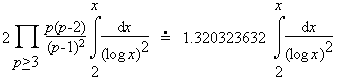# twin prime conjecture

The (weak) twin prime conjecture is that there are infinitely many twin primes.

There is also a strong form of this conjecture [HL23] which states that there are abouttwin primes less than or equal to x. The constant written above as an infinite product is the twin primes constant. See the page "a simple heuristic" linked below for information on how this conjecture is formed.